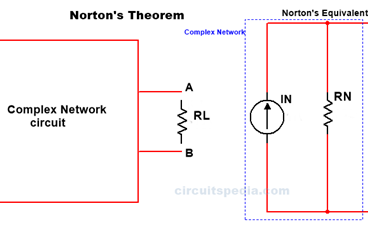;#### Norton theorem

Norton theorem Norton's theorem statement In Thevenin theorem, we see that in any complex circuit sources are converted in single voltage source Vth with the Series resistor Rth. Norton's theorem is also a method to convert any complex network into a simple circuit. Norton Theorem, any two-terminal complicated network circuit can be converted in single Current source IN in parallel […]